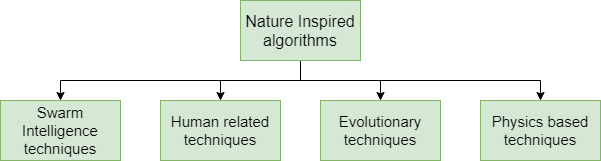GeeksforGeeks App
Open AppBrowser
Continue

# Dragon fly Optimization

Due to its simplicity, easy operation, capacity to protect against local optima, and the problem of derivatives free, Metaheuristic was frequently employed throughout the previous three decades. Exploration and exploitation are two fundamental metaheuristic features. The first one shows how the algorithm is capable of detecting new search areas, while the second one concentrates on finding the optimal solution is a promising search area. The metaheuristic success is one that can balance exploration with exploitation.

There are several classifications for metaheuristics.

• Nature-inspired vs. non-nature inspired
• Population-based vs. single point search
• Dynamic objective function vs. static objective function
• One single neighborhood vs. various neighborhood
• Memory usage vs. memoryless methods

Nature-inspired classification, however, is widely used. Nature-inspired classification could be further categorized into four categories  Swarm Intelligence techniques,  Human related techniques, Evolutionary techniques, and Physics-based techniques.Some examples of these nature-inspired algorithms are Henry gas solubility optimization, Cat Swarm Optimization, Teaching Learning based Optimization, etc.

In this article, we shall discuss Dragonfly optimization which is a swarm intelligence optimization technique.

## Inspiration

Dragonfly optimization has static, dynamic phases analogous to exploration, exploitation respectively in metaheuristic optimization. In the static phase, Dragonflies create sub-swarms and fly over different areas. In the dynamic phase, dragonflies fly in bigger swarms and along one direction. According to a research paper published by Reynolds in 1987 Flocks, herds and schools: a distributed behavioral model a swarm follows three basic phases:

1. Separation: refers to the minimization of static collisions between entities in the vicinity of others.
2. Alignment: refers to entities matching speed with the speed of other local entities.
3. Cohesion: refers to the tendency of entities towards the center of the mass of the neighborhood.

Apart from these mentioned principles survival is vital in any swarm which is basically of two forms: being attracted to food sources and repelled from their natural predator.

## Mathematical Model

Let us define the principles mentioned in the inspiration section for dragonfly optimization.

Separation:where X, Xj denotes the position of the current individual, jth neighboring individual respectively, and N is the number of neighboring individuals.

Alignment:where Vj shows the velocity of jth neighboring individual

Cohesion:where X, Xj denotes the position of the current individual, jth neighboring individual respectively, and N is the number of neighboring individuals.

Attraction:Where X, X+ denote the position of the current individual, food source respectively.

Repulsion:Where X, X denotes the position of the current individual, natural predator respectively.

Two vectors are considered to update the position of artificial dragonflies in a search space and simulate their movements:

• Step vector ()
• Position vector ().

The step vector denotes the direction of the movement of the dragonflies and defined below as   (1):where s, a, c, f, r are the weights for their respective phases i.e. separation, alignment, cohesion, attraction, repulsion, w is the inertia weight and t is the iteration counter.

Altering the values of these weights exploration and exploitation phases can be achieved.

The position vector is simply calculated using step vector as   (2):For the exploration phase, low cohesion weight (c) and high alignment weight (a) are assigned, similarly for exploitation low alignment weight (a) and high cohesion weight (c) is assigned.

The position vector gives the position of the dragonfly however when there are no neighboring solutions the dragonflies are required to fly in random search space and their position is updated using the modified equation for position vector   (3):where levy(d) is:where r1, r2 range in [0,1], \beta is constant, \sigma calculated as follows:## Algorithm

Initialize the dragonfly population Xi  and their respective step vectosfor i=1,2,…,n

While iteration is not over or optimized result not achieved

• Calculate the fitness functions for each dragonfly
• Update the weights s, a, c, f, r, w and food sources, natural predator
• Calculate Separation (S), Alignment (A), Cohesion (C), Attraction (F) and repulsion (R)
• using their equations
• If Neighbor(dragonfly)1
• Update velocity vector () and position vector () using equations 1 and 2
• Else
• Update position vector using equation 3

End While

That is how dragonfly optimization works

References:

My Personal Notes arrow_drop_up